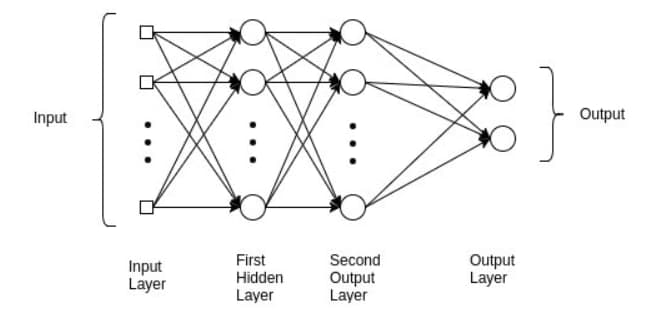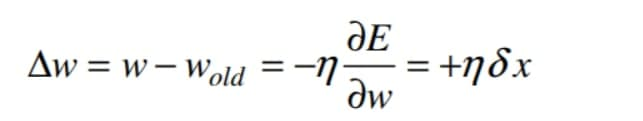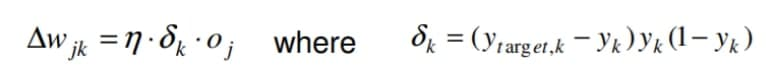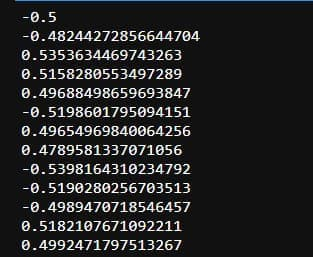## Multi-Layer Perceptron (MLP)

Anyone building a model in traditional Machine Learning has to either be an expert in the domain they are working on, or team up with one. It becomes increasingly challenging to design and engineer features without the expertise of this field. It is not only the quality of the dataset that determines the quality of the Machine Learning model. It is also how well the features encode the patterns in the dataset.Artificial Neural Networks form the basis of most Deep Learning algorithms. In contrast to other algorithms, they require no expert input during the development of features and engineering phases. NNs are able to determine characteristics of the data on their own. As Deep Learning algorithms analyze and identify patterns in the dataset, they learn which features to extract in order to visualize the data. Each representation of the dataset identifies a specific pattern or characteristic, and they are combined into a more abstract, high-level representation of the dataset . Due to this hands-off approach, algorithms can adapt a lot faster to the data at hand, since they do not require as much human involvement during the feature extraction and design process.

The structure of the brain can be inspired by neural networks, but the model does not have to be exact. Despite the fact that we still don’t fully understand how the brain works, the way it develops intelligence has served as inspiration for many scientific fields. Despite the existence of neural networks that were created to understand how brains work, Deep Learning as it stands today does not strive to replicate the brain’s functions.

The goal of Deep Learning, instead, is to enable systems to learn multiple levels of pattern compositions. Furthermore, just as with any scientific advance, Deep Learning didn’t start out with the complex structures and widespread applications that you see in recent publications. In order to address this limitation, the Multilayer Perceptron was developed. Non-linear neural networks are those whose inputs and outputs are not linearly mapped. Several layers of input and output and hidden layers with many neuronal stacks are included in a Multilayer Perceptron.

Multilayer Perceptrons rely on arbitrary activation functions rather than a threshold-imposing activation function like the Perceptron. In feedforward algorithms, the Multilayer Perceptron falls into the category of input-weighted sums with activation functions, just like the Perceptron is a feedforward algorithm. This differs from a linear combination in that each is propagated to a subsequent layer. The results of each layer are fed to the next based on their internal representations of the data. Similarly, the output is based on the results of the hidden layers. However, there’s more to it.

This algorithm cannot learn the weights that minimize the cost function if it only computes weighted sums in each neuron. It propagates the results to the output layer, and then stops there. There would be no actual learning if the algorithm calculated only one iteration. The Multilayer Perceptron expands horizons, increasing the number of layers of neurons a neural network can have, allowing it to learn more complex patterns.

## What is MLP

In neural networks, perceptron are the basic building blocks. Together, they form the basic layer of the neural network, in which each input is considered as an input, and some of the outputs are used as inputs for the next hidden layer. Usually non-linear complex problems are handled by neural networks, which are made up of multiple perceptron By combining hypotheses of single layer perceptron which add up to multilayer perceptron, we can manipulate the weights of those single layer perceptron to obtain the desired output, which is then fed as input to multilayer perceptron with new weights and activation functions. Consider the following scenario for layers that are known as hidden layers and work as a chain reaction.

When the data is in a nonlinear format, the effects of Multi-Layer perceptron can be seen. Since non-linearity data does not contain linearly labeled inputs and outputs, in fact, they are just randomly assigned.

## Why do we need MLP

In the end, the question arises as to why we felt we needed a multilayer perceptron when we already had a single layer perceptron. The basic answer to these questions involve a number of scenarios, some of which include huge amounts of data produced over the past several years, humans tending to solve complex tasks similar to their own minds, faster computations, and better scalability.

The multilayer perceptron opens up a world of possibilities to solve problems, and its functionality is so deep that it is beyond human understanding, just as the human mind is beyond our comprehension. A mind blowing MLP strategy that provides you with incredible predictions is offered.

MLPs are used when the data are not in a linear state or are not properly labeled. MLPs determine if the input data and the output data have similar functionality. The complexity of problems generated today requires human level thinking and the ability to make decisions accordingly, but this is not an easy task. In order to adapt and find the relation between linear and nonlinear data, we have to have a clear set of thoughts and operations. In this process, massive amounts of clean, stable data are used to train models. In order to mimic the functionality of a human brain, the multilayer perceptron is used to train the model. The concept is used almost in every industry and will most certainly be applied in the future if it is not currently being used. It performs some tasks better than humans and with more perfection than humans because it possesses human-level skills.

It has the ability to adapt to circumstance and to learn on its own so that it can provide maximum productivity, which is the need of the hour.

## How it actually worksMulti layer perceptron model consist of basically three layers and they are as follows

Input Layer: This layer consists of the input data that is already given and is decompressed in terms of smallest units called perceptron. Those perceptron then consist of some pieces of data which are vertically arranged and of all the inputs some perceptron are assigned to it. At the end all the perceptron are combined together and arranged to form the input layers. Let’s talk about the output layer and then we will move to the hidden layers

The output layer is the final layer which is generated post hidden layers. The data in the output layers are the results from the hidden layers. Now the input data does processing according to the given algorithms and methods with activation functions associated with hidden layers. In the end the output layer a series of final results are predicted and from those perceptrons the similar perceptron which is closest to output data(already provided) is finally predicted.

The hidden layer:The working of hidden layers is actually hidden just like the working of our minds so to put some light on to the process we will consider some scenarios in which the hidden layer might work.

There is a training pattern in which the model is trained. Let’s see how it happens.

First we Set up the network with N input units fully connected to M hidden nonlinear hidden units via connections with weights wij, which than are connected to P output units via connections with weights wjk

Now let’s Generate random initial weights. Than lets Select error function E(wjk) and learning rate η

Apply the updated weights equation ∆wjk=-η∂E(wjk)/∂wjk to each weight

wjk for each training pattern p.

Repeat the same for all hidden layers. Repeat steps until the network error function stops getting smaller.

Fine tuning weights
Generally, weight change from any unit j to unit k by gradient descent is now called Generalized Delta Rule or Backpropagation.Now, the weight change from the input layer unit i to hidden layer unit j is:The weight change from the hidden layer unit j to the output layer unit k is:Feed Forward Network, is a widely used  typical neural network method. Its target is to approximate some function f ().

Given, for example, a classifier y = f ∗ (x) that maps an input x to an output class y, the MLP find the best approximation to that classifier by defining a mapping, y = f(x; θ) and learning the best parameters θ for it.

The MLP networks consist of many functions that are chained together. A network with three layers would form f(x) = f (3)(f (2)(f (1)(x))).

All these layers are composed of units that perform transformation of a linear sum of inputs. The layer is represented as y = f(WxT + b).

Where f is the activation function (covered below), W is the set of weights, in the layer, x is the input vector, which can also be the output of the previous layer, and b is the bias vector.

weight = weight + learning rate * (expected – predicted) * x

## Code

We have imported all the necessary libraries which will define a function which than initialized the learning rate for the randomly generated values. The variables are declared with respect to the weights assigned.

				
// all the required libraries has been imported.

%use s2 // use this magic word or program might not run
import java.util.*
import kotlin.jvm.JvmStatic


				
class NeuralNetXor {
val ran = Random()
val eta = 0.3 //we have initialised the learning rate here

//to h1
var w1 = 0.0
var w2 = 0.0

//to h2
var w3 = 0.0
var w4 = 0.0

//to o1
var w5 = 0.0
var w6 = 0.0

//h1 b
var b1 = 0.0

//h2 b
var b2 = 0.0

//o1
var b3 = 0.0



Here we are passing a function which converting datatypes boolean values to double with if statement.

				
fun boolTdouble(in: Boolean): Double {
return if (in) {
1.0
} else {
0.0
}
}



The Neural Network function has been declared where two inputs are been provided with desired output as data. In the next section we are following typical feed forward method to train the model right from input layer upto the output layer while going through hidden layers.

				
// The Neural 2*2*1
// neural(input1,input2,desire output)
fun neural(x1: Double, x2: Double, d: Double): Double {
//forward approach
//from input layer to hidden layer
val n1 = w1 * x1 + w2 * x2 + b1
val n2 = w3 * x1 + w4 * x2 + b2
val h1 = 1 / (1 + Math.pow(Math.E, -1 * n1))
val h2 = 1 / (1 + Math.pow(Math.E, -1 * n2))
//hidden layer -&gt; output layer
val n3 = w5 * h1 + w6 * h2 + b3
val o = 1 / (1 + Math.pow(Math.E, -1 * n3))
//backward approach
//from output layer to hidden layer
w5 += eta * (d - o) * o * (1 - o) * h1
w6 += eta * (d - o) * o * (1 - o) * h2
b3 += eta * (d - o) * o * (1 - o) * 1
//from hidden layer to input layer
w1 += eta * (d - o) * o * (1 - o) * w5 * h1 * (1 - h1) * x1
w2 += eta * (d - o) * o * (1 - o) * w5 * h1 * (1 - h1) * x2
w3 += eta * (d - o) * o * (1 - o) * w6 * h2 * (1 - h2) * x1
w4 += eta * (d - o) * o * (1 - o) * w6 * h2 * (1 - h2) * x2
b1 += eta * (d - o) * o * (1 - o) * w5 * h1 * (1 - h1) * 1
b2 += eta * (d - o) * o * (1 - o) * w6 * h2 * (1 - h2) * 1
return d - o
}



Here we are training the module based on different functions for the appropriate convergence.

				
// learn AND
// this block will execute convergence
fun neuralAND() {
val x = ran.nextBoolean()
val y = ran.nextBoolean()
val z = x and y
println(neural(boolTdouble(x), boolTdouble(y), boolTdouble(z)))
}

// learn OR
// this block will execute convergence
fun neuralOR() {
val x = ran.nextBoolean()
val y = ran.nextBoolean()
val z = x or y
println(neural(boolTdouble(x), boolTdouble(y), boolTdouble(z)))
}

// learn XOR
// this block will execute convergence
fun neuralXOR() {
val x = ran.nextBoolean()
val y = ran.nextBoolean()
val z = x xor y
println(neural(boolTdouble(x), boolTdouble(y), boolTdouble(z)))
}



At last we are assigning the weights to specific function and initializing the network.

				
// weights are being initialized
// random 0~1
fun init() {
w1 = ran.nextDouble()
w2 = ran.nextDouble()
b1 = ran.nextDouble()
}
}



At last as you can see we have declared a variable which contains values from above functions and generating the result with for statement.

				
val neural = NeuralNetXor()
neural.init()
for (i in 0..299999) {
neural.neuralXOR()
}



## Output## Limitations

Let’s consider a scenario where we face the most common issue of high residual which is the cost function. If we receive the output as is not desired then we won’t be able to figure out what’s the problem that makes the hidden layers make this decision as they are responsible for the output layer. So this is one of the limitations that needs to be taken care of and it is also taken care of by backpropagation. More on backpropagation later but as of now let me just tell you that this method of approach where the cost function is analyzed and then the input is feeded to the output layers based on the actual output. Hence in simple words the hidden layer is told to make changes to the weights according to the output and then the whole process is initiated.

## Conclusion

The MLP is used worldwide according to the needs which reflects its importance and performance but according to me its peak is yet to come since this is only the initial step of Artificial Intelligence there is a lot more to come in the future.

That’s it for the topic. I hope you have learned something new and invested your time in the right place.